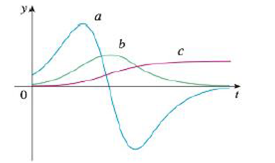Chapter 2.8, Problem 51E

Chapter
Section
Textbook Problem

The figure shows the graphs of three functions. One is the position function of a car, one is the velocity of the car, and one is its acceleration. Identify each curve, and explain your choices.To determine

To identify: Each curves on the given graph and give proper explanation.

Explanation

Graph:

The given graph is shown as in Figure 1,

Observation:

Observe the graph of b and a carefully.

The point where a(t)=0 is the same point where graph of b(t) has horizontal tangent.

Recall that the derivative of a function is zero where the function has a horizontal tangent.

Therefore, a(t) is the derivative of the graph b(t). That is, b(t)=a(t).

Observe the graph of a and c carefully

Still sussing out bartleby?

Check out a sample textbook solution.

See a sample solution

The Solution to Your Study Problems

Bartleby provides explanations to thousands of textbook problems written by our experts, many with advanced degrees!

Get Started

In Problems 17-24, find the slope and y-intercept and then graph each equation.

Mathematical Applications for the Management, Life, and Social Sciences

True or False: converges.

Study Guide for Stewart's Multivariable Calculus, 8th

Identify the basic features of a between-subjects research design.

Research Methods for the Behavioral Sciences (MindTap Course List)

Find each value. log39

College Algebra (MindTap Course List)